# 基础数据结构 例：栈、队列、链表、数据、字典、树、等

2020/02/14 16:17

### 栈 stack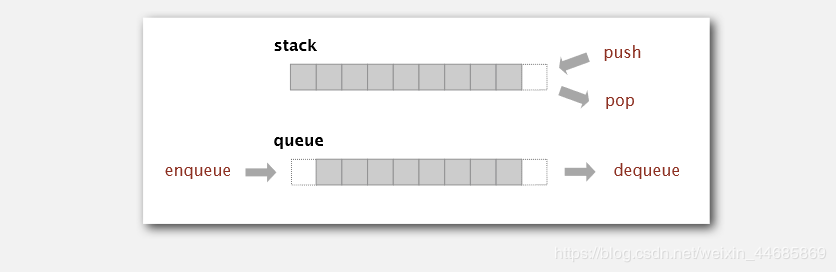Python实现

# 栈的顺序表实现

class Stack(object):
def __init__(self):
self.items = []

def isEmpty(self):
return self.items == []

def push(self, item):
return self.items.append(item)

def pop(self):
return self.items.pop()

def top(self):
return self.items[len(self.items)-1]

def size(self):
return len(self.items)

if __name__ == '__main__':
stack = Stack()
stack.push("Hello")
stack.push("World")
stack.push("!")
print(stack.size())
print(stack.top())
print(stack.pop())
print(stack.pop())
print(stack.pop())

# 结果
3
!
!
World
Hello
# 栈的链接表实现

class SingleNode(object):
def __init__(self, item):
self.item = item
self.next = None

class Stack(object):
def __init__(self):

def isEmpty(self):

def push(self, item):
node = SingleNode(item)

def pop(self):
return cur.item

def top(self):

def size(self):
count = 0
while cur != None:
count += 1
cur = cur.next
return count

if __name__ == '__main__':
stack = Stack()
stack.push("Hello")
stack.push("World")
stack.push("!")
print(stack.size())
print(stack.top())
print(stack.pop())
print(stack.pop())
print(stack.pop())

# 结果
3
!
!
World
Hello 

### 队列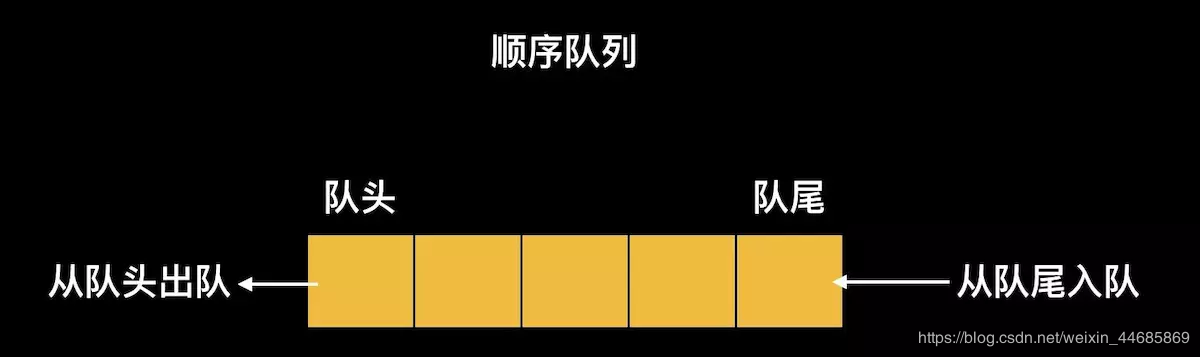class Queue:
"""

"""

def __init__(self):
"""

"""
self.__queue = []

def first(self):
"""

:return:如果队列为空，则返回None，否则返回第一个元素
"""
return None if self.isEmpty() else self.__queue

def enqueue(self, obj):
"""

:param obj:要加入队列的对象
"""
self.__queue.append(obj)

def dequeue(self):
"""

:return:如果队列为空，则返回None，否则返回dequeued元素
"""
return None if self.isEmpty() else self.__queue.pop(0)

def clear(self):
"""

"""
self.__queue.clear()

def isEmpty(self):
"""

"""
return self.length() == 0

def length(self):
"""

"""
return len(self.__queue)

class PriorQueue:
"""

"""

def __init__(self, objs=[]):
"""

:参数objs:对象列表初始化
"""
self.__prior_queue = list(objs)
# 排序从最大值到最小值，最小值具有最高的优先级
# 使得“dequeue”的效率为O(1)
self.__prior_queue.sort(reverse=True)

def first(self):
"""

:return:如果优先队列为空，则返回None，否则返回优先级最高的元素
"""
return None if self.isEmpty() else self.__prior_queue[-1]

def enqueue(self, obj):
"""

:param obj:要加入队列的对象
"""
index = self.length()
while index > 0:
if self.__prior_queue[index - 1] < obj:
index -= 1
else:
break
self.__prior_queue.insert(index, obj)

def dequeue(self):
"""

:return:如果优先队列为空，则返回None，否则返回dequeued元素
"""
return None if self.isEmpty() else self.__prior_queue.pop()

def clear(self):
"""

"""
self.__prior_queue.clear()

def isEmpty(self):
"""

"""
return self.length() == 0

def length(self):
"""

"""
return len(self.__prior_queue)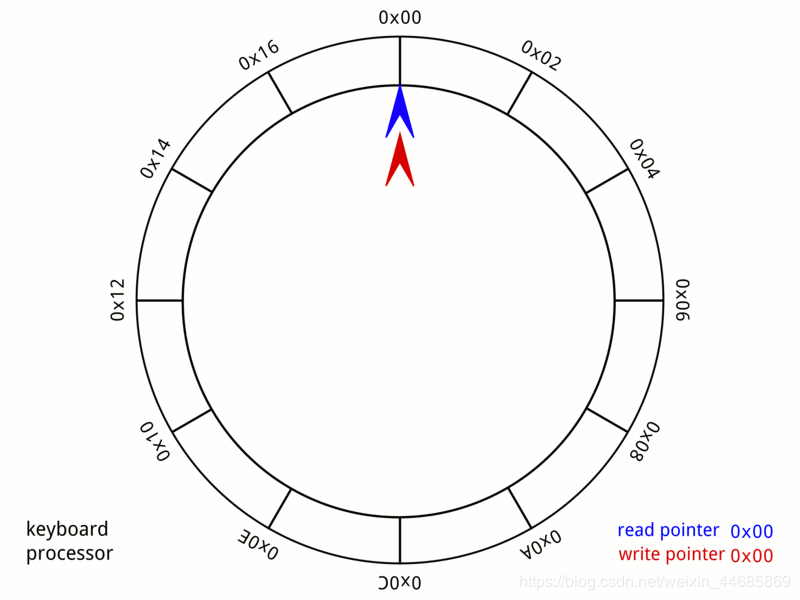class Loopqueue:
'''

'''
def __init__(self, length):
self.tail = 0
self.maxSize = length
self.cnt = 0
self.__list = [None]*length

def __len__(self):
'''

'''
return self.cnt

def __str__(self):
'''

'''
s = ''
for i in range(self.cnt):
index = (i + self.head) % self.maxSize
s += str(self.__list[index])+' '
return s

def isEmpty(self):
'''

'''
return self.cnt == 0

def isFull(self):
'''

'''
return self.cnt == self.maxSize

def push(self, data):
'''

'''
if self.isFull():
return False
if self.isEmpty():
self.__list = data
self.tail = 0
self.cnt = 1
return True
self.tail = (self.tail+1)%self.maxSize
self.cnt += 1
self.__list[self.tail] = data
return True

def pop(self):
'''

'''
if self.isEmpty():
return False
self.cnt -= 1
return data

def clear(self):
'''

'''
self.tail = 0
self.cnt = 0
return True

2个附加操作：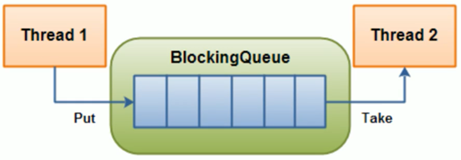import threading #引入线程 上锁
import time
from collections import deque # 导入队列

class BlockQueue:
def __init__(self, maxsize=0):
'''

'''
self.maxsize = maxsize
self.unfinished = 0

'''

'''
unfinish = self.unfinished - 1
if unfinish <= 0:
if unfinish < 0:
raise ValueError("The number of calls to task_done() is greater than the number of queue elements")
self.unfinished = unfinish

def join(self):
'''

'''
while self.unfinished:

def put(self, item, block=True, timeout=None):
'''
block=True一直阻塞直到有一个空闲的插槽可用，n秒内阻塞，如果在那个时间没有空闲的插槽，则会引发完全的异常。
Block=False如果一个空闲的槽立即可用，则在队列上放置一个条目，否则就会引发完全的异常（在这种情况下，“超时”将被忽略）。

'''
with self.not_full:
if self.maxsize > 0:
if not block:
if self._size() >= self.maxsize:
raise Exception("The BlockQueue is full")
elif timeout is not None:
self.not_full.wait()
elif timeout < 0:
raise Exception("The timeout period cannot be negative")
else:
endtime = time.time() + timeout
while self._size() >= self.maxsize:
remaining = endtime - time.time()
if remaining <= 0.0:
raise Exception("The BlockQueue is full")
self.not_full.wait(remaining)
self.queue.append(item)
self.unfinished += 1
self.not_empty.notify()

def get(self, block=True, timeout=None):
'''

'''
with self.not_empty:
if not block:
if self._size() <= 0:
raise Exception("The queue is empty and you can't call get ()")
elif timeout is None:
while not self._size():
self.not_empty.wait()
elif timeout < 0:
raise ValueError("The timeout cannot be an integer")
else:
endtime = time.time() + timeout
remaining = endtime - time.time()
if remaining <= 0.0:
raise Exception("The BlockQueue is empty")
self.not_empty.wait(remaining)
item = self._get()
self.not_full.notify()
return item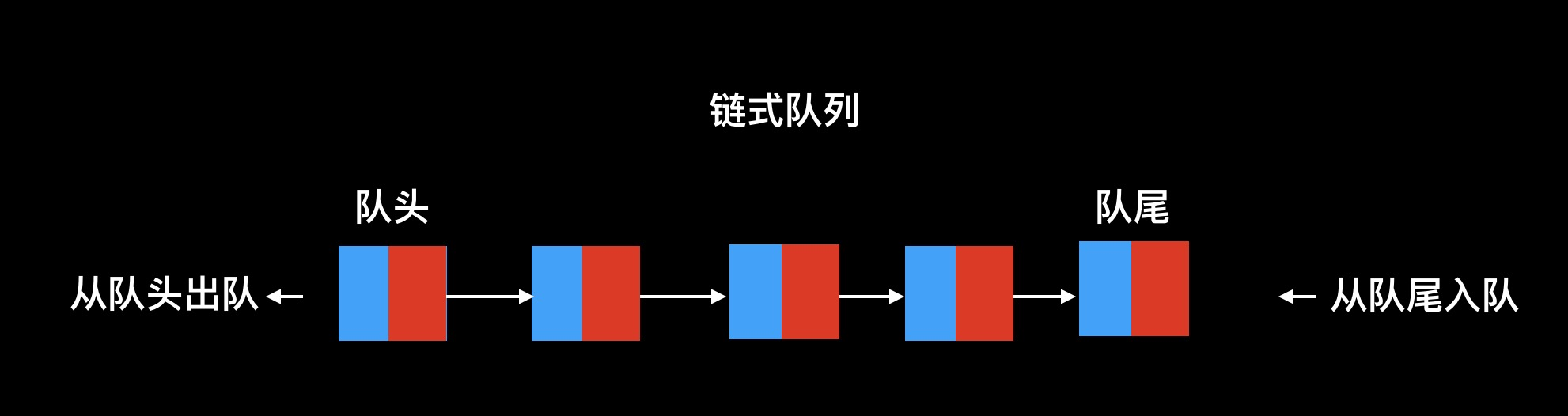class QueueNode():
def __init__(self):
self.data = None
self.next = None
def __init__(self):
tQueueNode = QueueNode()
self.front = tQueueNode
self.rear = tQueueNode
'''判断是否为空'''
def IsEmptyQueue(self):
if self.front == self.rear:
iQueue = True
else:
iQueue = False
return iQueue
'''进队列'''
def EnQueue(self,da):
tQueueNode = QueueNode()
tQueueNode.data = da
self.rear.next = tQueueNode
self.rear = tQueueNode
print("当前进队的元素为：",da)
'''出队列'''
def DeQueue(self):
if self.IsEmptyQueue():
print("队列为空")
return
else:
tQueueNode = self.front.next
self.front.next = tQueueNode.next
if self.rear == tQueueNode:
self.rear = self.front
return tQueueNode.data
if self.IsEmptyQueue():
print("队列为空")
return
else:
return self.front.next.data
def CreateQueueByInput(self):
data = input("请输入元素（回车键确定，#结束）")
while data != "#":
self.EnQueue(data)
data = input("请输入元素（回车键确定，#结束）")
'''遍历顺序队列内的所有元素'''
def QueueTraverse(self):
if self.IsEmptyQueue():
print("队列为空")
return
else:
while self.front != self.rear:
result = self.DeQueue()
print(result,end = ' ')
lq.CreateQueueByInput()
print("队列里元素为：")
lq.QueueTraverse()

# 结果

5 8 9 

### 链表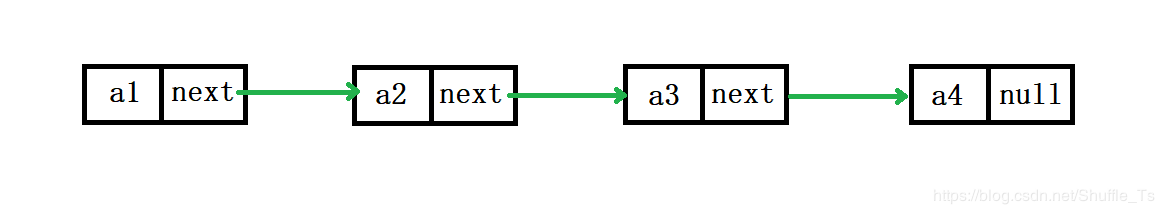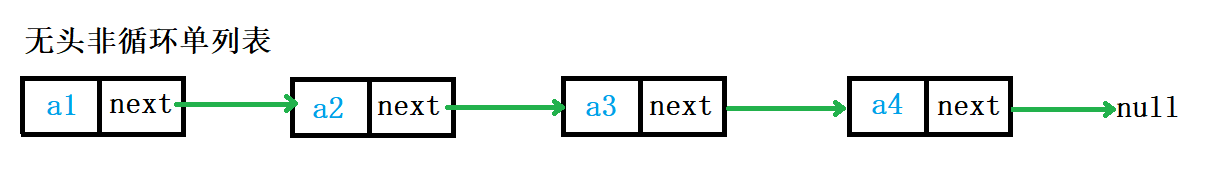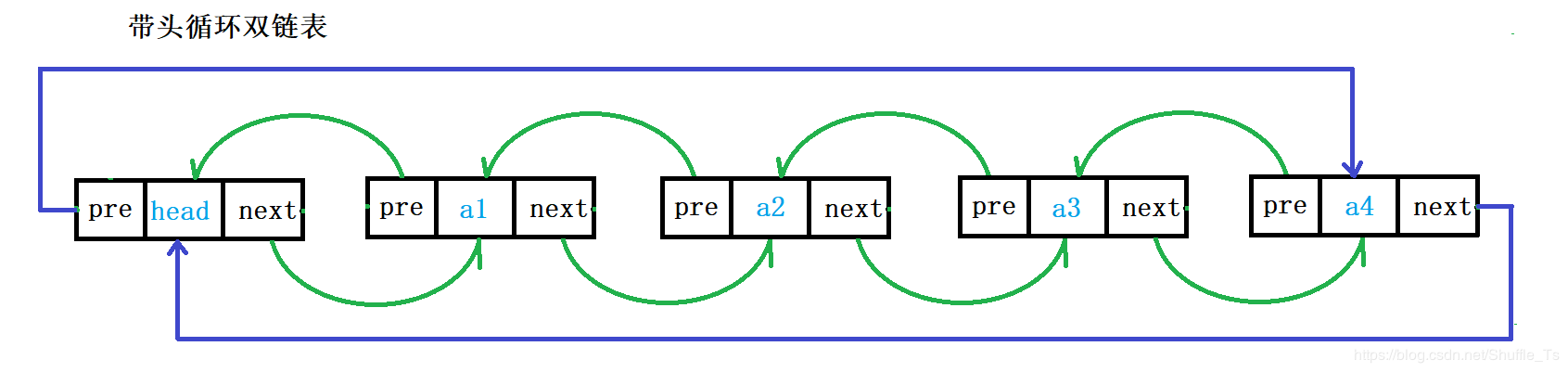带头双向循环链表：结构最复杂，一般单独存储数据。实际中经常使用的链表数据结构，都是带头双向循环链表。这个结构虽然复杂，但是使用代码实现后会发现这个结构会带来很多优势，实现反而简单了。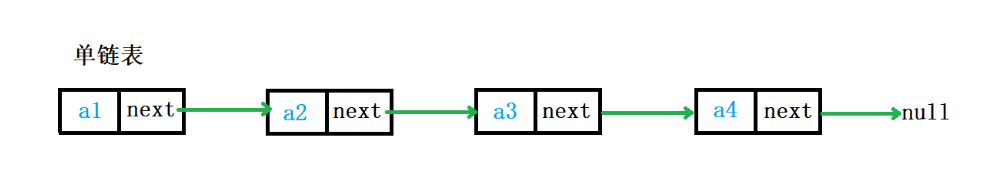class Node(object):
"""节点"""

def __init__(self, elem):
self.elem = elem
self.next = None # 初始设置下一节点为空

'''

'''

# 下面创建单链表，并实现其应有的功能

"""单链表"""

def __init__(self, node=None): # 使用一个默认参数，在传入头结点时则接收，在没有传入时，就默认头结点为空

def is_empty(self):
'''链表是否为空'''

def length(self):
'''链表长度'''
# cur游标，用来移动遍历节点
# count记录数量
count = 0
while cur != None:
count += 1
cur = cur.next
return count

def travel(self):
'''遍历整个列表'''
while cur != None:
print(cur.elem, end=' ')
cur = cur.next
print("\n")

'''链表头部添加元素'''
node = Node(item)

def append(self, item):
'''链表尾部添加元素'''
node = Node(item)
# 由于特殊情况当链表为空时没有next，所以在前面要做个判断
if self.is_empty():
else:
while cur.next != None:
cur = cur.next
cur.next = node

def insert(self, pos, item):
'''指定位置添加元素'''
if pos <= 0:
# 如果pos位置在0或者以前，那么都当做头插法来做
elif pos > self.length() - 1:
# 如果pos位置比原链表长，那么都当做尾插法来做
self.append(item)
else:
count = 0
while count < pos - 1:
count += 1
per = per.next
# 当循环退出后，pre指向pos-1位置
node = Node(item)
node.next = per.next
per.next = node

def remove(self, item):
'''删除节点'''
pre = None
while cur != None:
if cur.elem == item:
# 先判断该节点是否是头结点
else:
pre.next = cur.next
break
else:
pre = cur
cur = cur.next

def search(self, item):
'''查找节点是否存在'''
while not cur:
if cur.elem == item:
return True
else:
cur = cur.next
return False

if __name__ == "__main__":

# node = Node(100) # 先创建一个节点传进去

print(ll.is_empty())
print(ll.length())

ll.append(3)
ll.insert(-3, 110)
ll.insert(99, 111)
print(ll.is_empty())
print(ll.length())
ll.travel()
ll.remove(111)
ll.travel()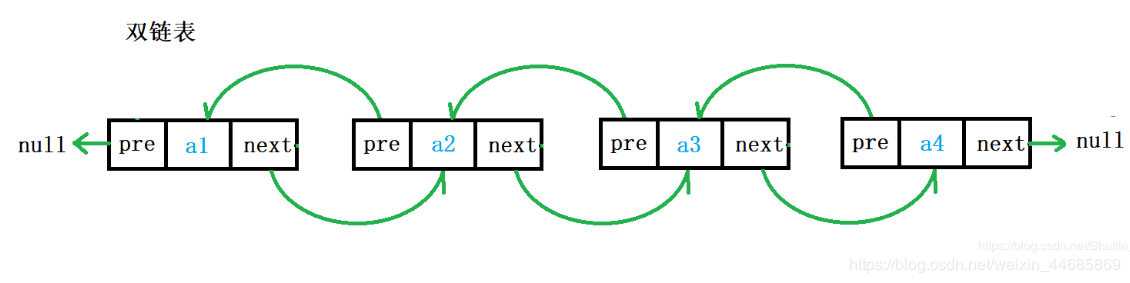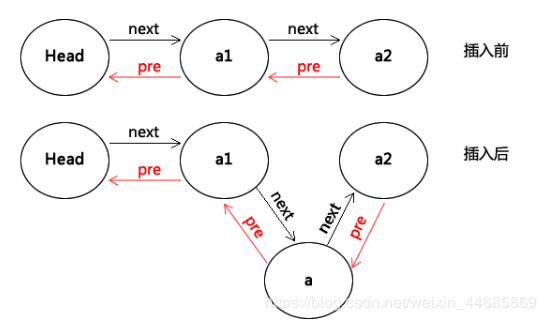class Node(object):
def __init__(self,val,p=0):
self.data = val
self.next = p
self.prev = p

def __init__(self):

def __getitem__(self, key):

if self.is_empty():
return

elif key <0 or key > self.getlength():
print 'the given key is error'
return

else:
return self.getitem(key)

def __setitem__(self, key, value):

if self.is_empty():
return

elif key <0 or key > self.getlength():
print 'the given key is error'
return

else:
self.delete(key)
return self.insert(key)

def initlist(self,data):

for i in data[1:]:
node = Node(i)
p.next = node
node.prev = p
p = p.next

def getlength(self):

length = 0
while p!=0:
length+=1
p = p.next

return length

def is_empty(self):

if self.getlength() ==0:
return True
else:
return False

def clear(self):

def append(self,item):

q = Node(item)
else:
while p.next!=0:
p = p.next
p.next = q
q.prev = p

def getitem(self,index):

if self.is_empty():
return
j = 0

while p.next!=0 and j <index:
p = p.next
j+=1

if j ==index:
return p.data

else:

print 'target is not exist!'

def insert(self,index,item):

if self.is_empty() or index<0 or index >self.getlength():
return

if index ==0:

j = 0
while p.next!=0 and j<index:
post = p
p = p.next
j+=1

if index ==j:
q = Node(item,p)
post.next = q
q.prev = post
q.next = p
p.prev = q

def delete(self,index):

if self.is_empty() or index<0 or index >self.getlength():
return

if index ==0:

j = 0
while p.next!=0 and j<index:
post = p
p = p.next
j+=1

if index ==j:
post.next = p.next
p.next.prev = post

def index(self,value):

if self.is_empty():
return

i = 0
while p.next!=0 and not p.data ==value:
p = p.next
i+=1

if p.data == value:
return i
else:
return -1

l.initlist([1,2,3,4,5])
print l.getitem(4)
l.append(6)
print l.getitem(5)

l.insert(4,40)
print l.getitem(3)
print l.getitem(4)
print l.getitem(5)

l.delete(5)
print l.getitem(5)

l.index(5)

# 结果
5
6
4
40
5
6

查找时也是一样的，可以用二分法的思路，从头节点向后和尾节点向前同时进行，这样效率也可以提高一倍，但是为什么市场上对于单链表的使用要超过双链表呢？从存储结构来看，每一个双链表的节点都比单链表的节点多一个指针，如果长度是n，就需要n*lenght（32位是4字节，64位是8字节）的空间，这在一些追求时间效率不高的应用下就不适用了，因为他占的空间大于单链表的1/3，所以设计者就会一时间换空间。

1234->2134->2314->2341->3241->3421->4321,这样也太费劲了，类似冒泡排序了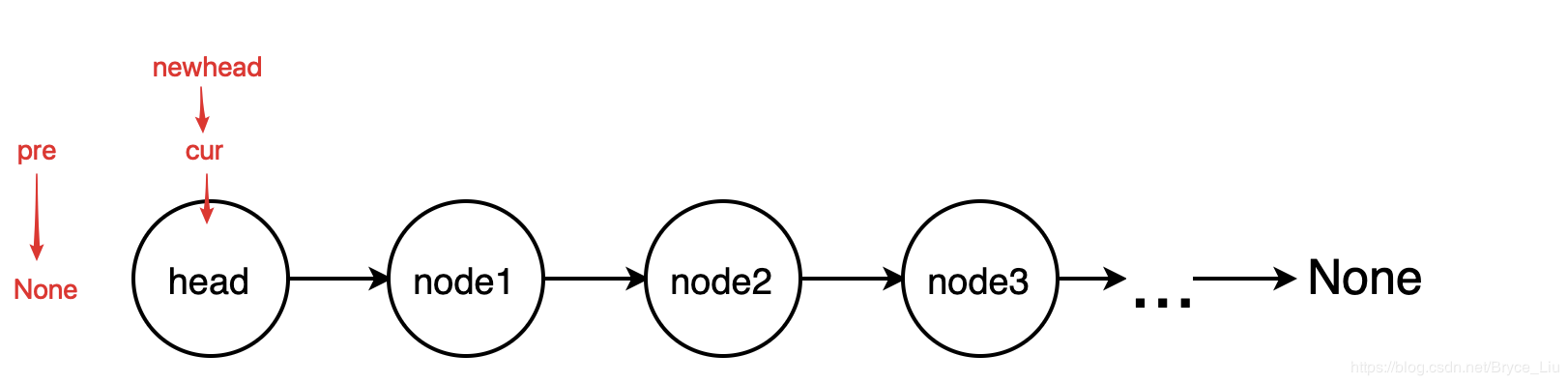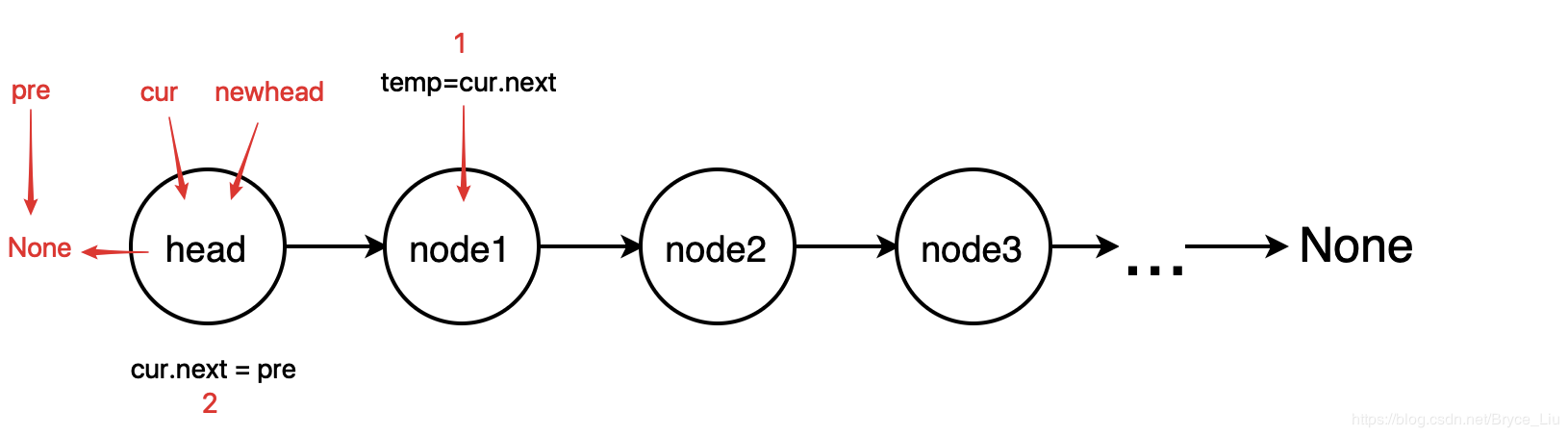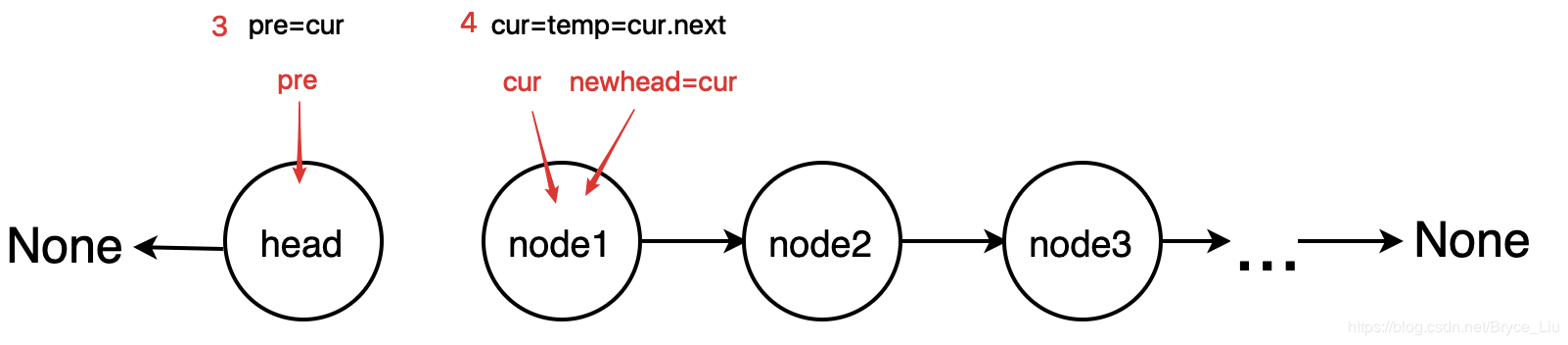class ListNode:
def __init__(self, x):
self.val = x
self.next = None

# 定义反转的初始状态
pre = None
while cur:
tmp = cur.next
cur.next = pre
pre = cur
cur = tmp

if __name__ == '__main__':
p1 = ListNode(2) # 建立链表1->2->3->4->None;
p2 = ListNode(3)
p3 = ListNode(4)
p1.next = p2
p2.next = p3
p = reverse(head) # 输出链表 4->3->2->1->None
while p:
print(p.val)
p = p.next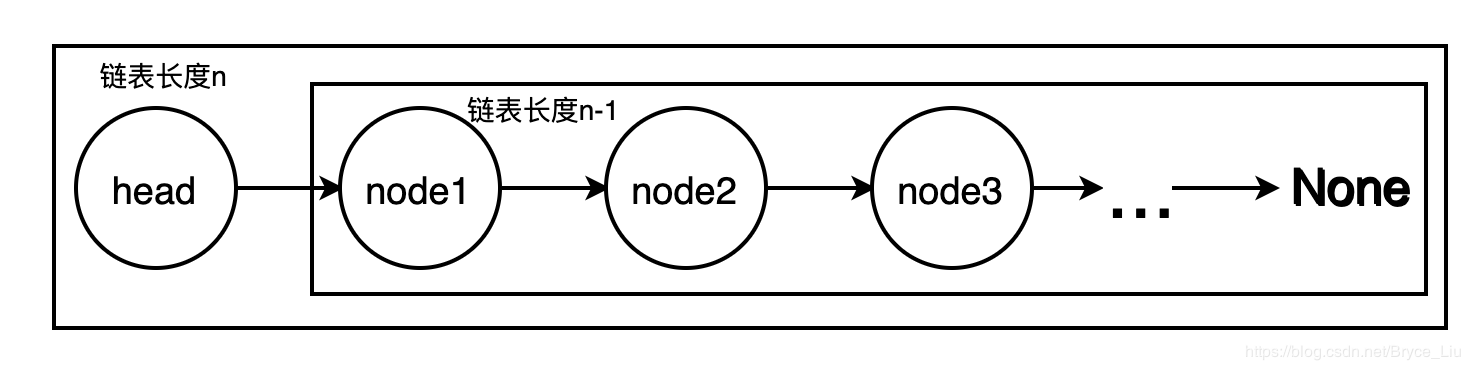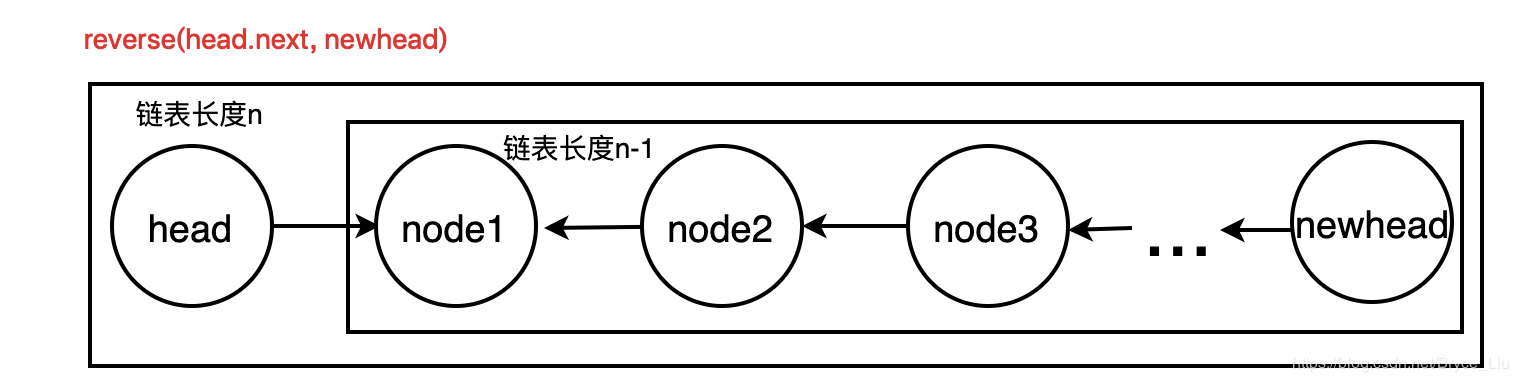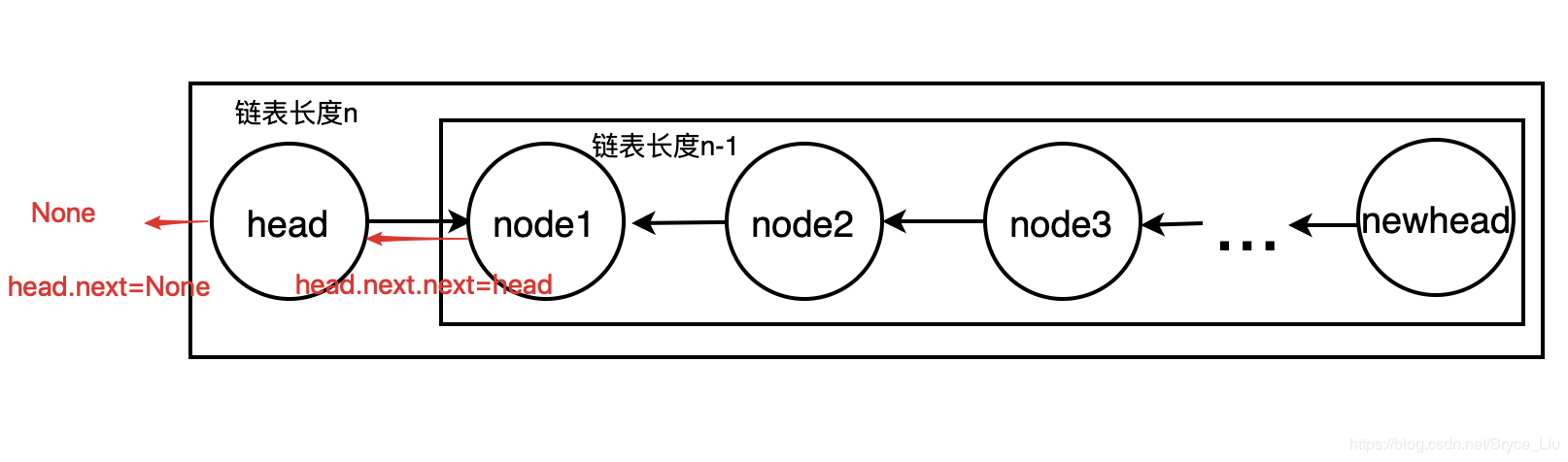class ListNode:
def __init__(self, x):
self.val = x
self.next = None

return
else:

if __name__ == '__main__':
p1 = ListNode(2) # 建立链表1->2->3->4->None
p2 = ListNode(3)
p3 = ListNode(4)
p1.next = p2
p2.next = p3
while p:
print(p.val)
p = p.next

### 数组

Python 没有内置对数组的支持，但可以使用 Python 列表代替。

int类型，该类型占两个字节的内存空间，所以每个元素均占有两个 字节（图中每一格为一字节）。

### 字典实现原理 NSDictionary

Python 中 dict 对象是表明了其是一个原始的Python数据类型，按照键值对的方式存储，其中文名字翻译为字典，顾名思义其通过键名查找对应的值会有很高的效率，时间复杂度在常数级别O(1)

dict底层实现

>>> map(hash, (0, 1, 2, 3))

[0, 1, 2, 3]

>>> map(hash, ("namea", "nameb", "namec", "named"))

[-1658398457, -1658398460, -1658398459, -1658398462]

1.根据 key 计算出它的哈希值 h。

2.假设箱子的个数为 n，那么这个键值对应该放在第 (h % n) 个箱子中。

3.如果该箱子中已经有了键值对，就使用开放寻址法或者拉链法解决冲突。

1.如果哈希表中本来箱子就比较多，扩容时需要重新哈希并移动数据，性能影响较大。

2.如果哈希函数设计不合理，哈希表在极端情况下会变成线性表，性能极低。

Python中所有不可变的内置类型都是可哈希的。

### 树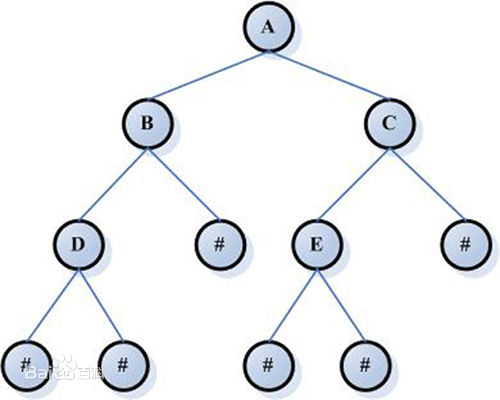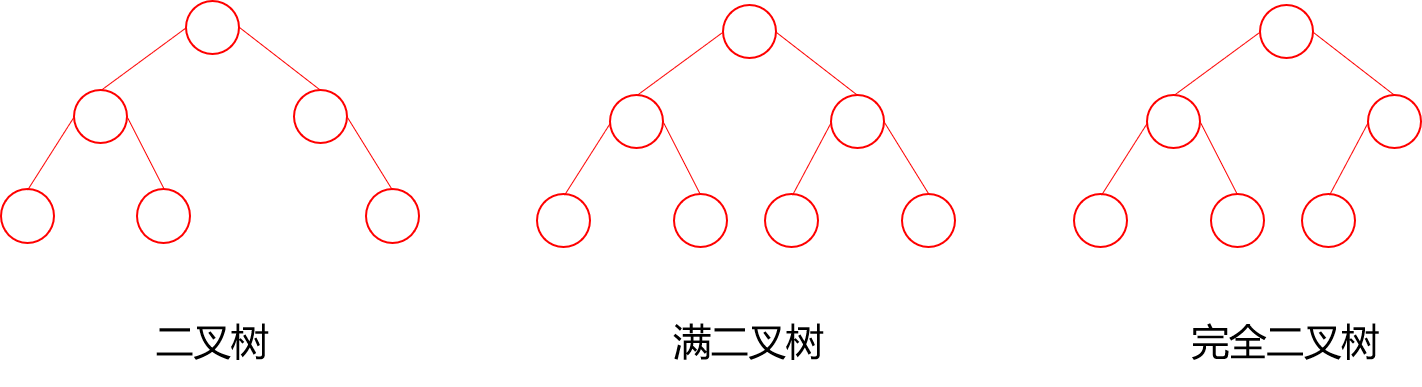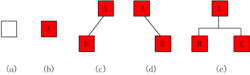(1)空二叉树——如图(a)；
(2)只有一个根结点的二叉树——如图(b)；
(3)只有左子树——如图(1)；
(4)只有右子树——如图(3)；
(5)完全二叉树——如图(3)。

hash树

### B-tree/B+tree

Btree
Btree是一种多路自平衡搜索树，它类似普通的二叉树，但是Btree允许每个节点有更多的子节点。Btree示意图如下：B+tree

B+树是B树的变体，也是一种多路平衡查找树，B+树的示意图为：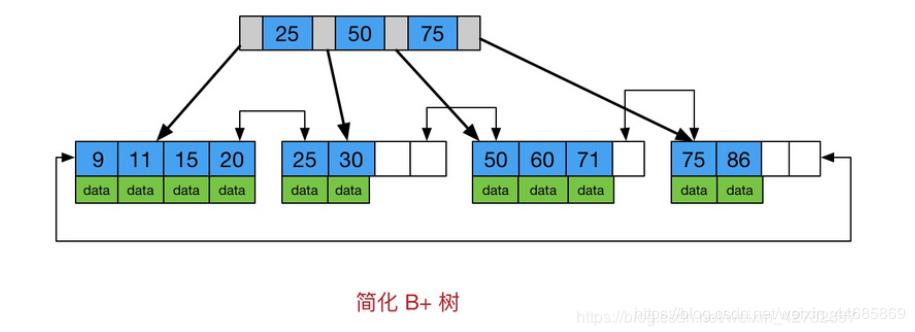### 作者的其它热门文章

0
0 收藏

0 评论
0 收藏
0